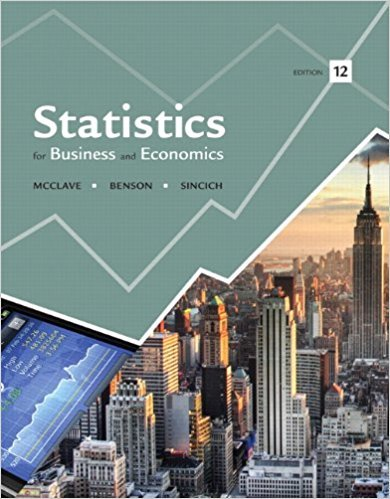×
Get Full Access to Statistics For Business And Economics - 12 Edition - Chapter 4 - Problem 54e
Get Full Access to Statistics For Business And Economics - 12 Edition - Chapter 4 - Problem 54e

×

# Making your vote count. Refer to the Chance (Fall 2007)ISBN: 9780321826237 51

## Solution for problem 54E Chapter 4

Statistics for Business and Economics | 12th Edition

• Textbook Solutions
• 2901 Step-by-step solutions solved by professors and subject experts
• Get 24/7 help from StudySoup virtual teaching assistantsStatistics for Business and Economics | 12th Edition

4 5 1 263 Reviews
22
4
Problem 54E

Problem 54E

Making your vote count. Refer to the Chance (Fall 2007) study on making your vote count, Exercise 3.27 (p. 143). Recall the scenario where you are one of five county commissioners voting on an issue, and each commissioner is equally likely to vote for or against.

a. Your vote counts (i.e., is the decisive vote) only if the other four voters split, two in favor and two against. Use the binomial distribution to find the probability that your vote counts.

b. If you convince two other commissioners to “vote in bloc” (i.e., you all agree to vote among yourselves first, and whatever the majority decides is the way all three will vote, guaranteeing that the issue is decided by the bloc), your vote counts only if these two commissioners split their bloc votes, one in favor and one against. Again, use the binomial distribution to find the probability that your vote counts.Step-by-Step Solution:

Solution :

Step 1 of 2:

Given one of 5 county commissioners voting on an issue, and each commissioner is equally likely to vote for or against.

Our goal is :

a). If the other four voters splits, then we need to find the probability that your vote counts.

b). If these two commissioners split their bloc votes, then we have to find the probability

a). Now we have to find the probability that your vote counts.

The number of commissioners out of 4 who vote in favour of an issue is x.

Given 2 in favour and 2 against.

So x=2.

Let x follows binomial distribution.

Then the probability function of x isWhere n = 4

The probability of each commissioner vote is

P(favor) = P(against)

P(favor) = 0.5 and

q = 1-p

q = 1-0.5

q = 0.5

Therefore, q=0.5.

Then the probability that your vote is equal to P(x=2).0.375

Therefore, the probability that your vote counts is 0.375.

Step 2 of 2

##### ISBN: 9780321826237

Unlock Textbook Solution